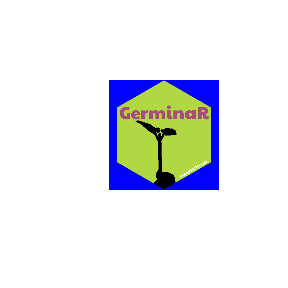# Sticker design

In the layer include_image(opts = "magick package") you can add different arguments and combine them using asterisk (*).

Options available in magick package

Select different panel color for your sticker (i.e. include_shape(panel_color = "color")).

library(huito)

font <- c("Paytone One", "Permanent Marker")

huito_fonts(font)

label <- label_layout(size = c(5.08, 5.08)
, border_width = 0
, background = "#b1d842"
) %>%
include_image(value = "https://germinar.inkaverse.com/img/seed_germination.png"
, size = c(5.5, 5.5)
, position = c(2.55, 1.26)
, opts = 'image_transparent("white")*image_modulate(brightness = 0)'
) %>%
include_shape(size = 5.08
, border_width = 0
, position = c(2.54, 2.54)
, panel_color = "blue"
) %>%
include_text(value = "GerminaR"
, font
, size = 23
, position = c(2.54, 3.55)
, color = "#a64d79"
) %>%
include_text(value = "inkaverse.com"
, font
, size = 6
, position = c(3.9, 0.96)
, angle = 30
, color = "white"
)

## Preview mode

label %>%
label_print(mode = "preview")## Complete mode

The final file is exported in pdf format.

sticker <- label %>%
label_print(filename = "GerminaR"
, margin = 0
, paper = c(5.5, 5.5)
, mode = "complete"
)